# Triangle IRT

An isosceles right triangle ABC with right angle at vertex C has vertex coordinates: A (-1, 2); C (-5, -2) Calculate the length of segment AB.

x =  8

### Step-by-step explanation: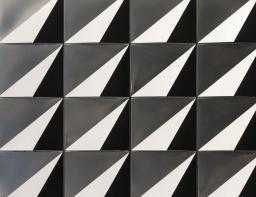Did you find an error or inaccuracy? Feel free to write us. Thank you!Tips to related online calculators
Line slope calculator is helpful for basic calculations in analytic geometry. The coordinates of two points in the plane calculate slope, normal and parametric line equation(s), slope, directional angle, direction vector, the length of the segment, intersections of the coordinate axes, etc.
Pythagorean theorem is the base for the right triangle calculator.

#### You need to know the following knowledge to solve this word math problem:

We encourage you to watch this tutorial video on this math problem:

## Related math problems and questions:

• Isosceles triangleIn an isosceles triangle ABC with base AB; A [3,4]; B [1,6] and the vertex C lies on the line 5x - 6y - 16 = 0. Calculate the coordinates of vertex C.
• The coordinates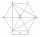The coordinates (5, 2) and (-6, 2) are vertices of a hexagon. Explain how to find the length of the segment formed by these endpoints. How long is the segment?
• Segment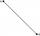Calculate the segment AB's length if the coordinates of the end vertices are A[10, -4] and B[5, 5].
• Coordinates of a centroindLet’s A = [3, 2, 0], B = [1, -2, 4] and C = [1, 1, 1] be 3 points in space. Calculate the coordinates of the centroid of △ABC (the intersection of the medians).
• Center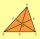In the triangle ABC is point D[1,-2,6], which is the center of the |BC|, and point G[8,1,-3], which is the center of gravity of the triangle. Find the coordinates of the vertex A[x,y,z].
• Rectangular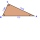Rectangular triangle KLM with right angle at vertex L, angle beta at vertex K and angle alpha at vertex M. Angle at vertex M = 65°, side l = 17.5 cm. Use Pythagorean theorems and trigonometric functions to calculate the lengths of all sides and the angle
• Right angleIn a right triangle ABC with a right angle at the apex C, we know the side length AB = 24 cm and the angle at the vertex B = 71°. Calculate the length of the legs of the triangle.
• Construct 8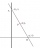Construct an analytical geometry problem where it is asked to find the vertices of a triangle ABC: the vertices of this triangle must be the points A (1,7) B (-5,1) C (5, -11). the said problem should be used the concepts of: distance from a point to a li
• IsoscelesIsosceles trapezium ABCD ABC = 12 angle ABC = 40 ° b=6. Calculate the circumference and area.
• A cell tower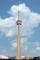A cell tower is located at coordinates (-5, -7) and has a circular range of 12 units. If Mr. XYZ is located at coordinates (4,5), will he be able to get a signal?
• Right isosceles triangleRight isosceles triangle has an altitude x drawn from the right angle to the hypotenuse dividing it into 2 equal segments. The length of one segment is 5 cm. What is the area of the triangle?
• Center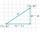Calculate the coordinates of the center of gravity T [x, y] of triangle ABC; A[11,4] B[13,-7] C[-17,-18].
• Right angled triangle 2LMN is a right-angled triangle with vertices at L(1,3), M(3,5), and N(6,n). Given angle LMN is 90° find n
• ABC isosceles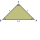ABC isosceles rights triangle the length or each leg is 1 unit what is the length of the hypotenuse AB in the exact form
• Main/central vertex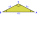ABC is an isosceles triangle with base BC and main vertex A. The angle at vertex A is 18°. What will be the size of the angle at vertex B?
• Medians and sidesTriangle ABC in the plane Oxy; are the coordinates of the points: A = 2.7 B = -4.3 C-6-1 Try calculate lengths of all medians and all sides.
• Internal anglesFind the internal angles of the triangle ABC if the angle at the vertex C is twice the angle at the B and the angle at the vertex B is 4 degrees smaller than the angle at vertex A.